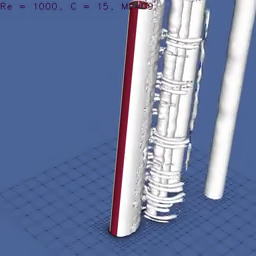# Encode a movie that displays octree data on an octree

Perhaps we can employ the wavelet-based feature-detection algorithm to encode spatiotemporal data on an octree grid. For this purpose we “compress” the data from N frames of size N \times N pixels.

Original data

Intensity + OctreeA slice of the octree data in “false color”

Movie of the octree false color data

## Is it efficient?

No, the dump file that encodes the octree data is about 200 MB. The original N \times N px movie (of 751 frames) was 1.9 MB in size. Even if te account for the unsigned char to double overhead, it does not really work. This motivates to store gridded data in an .mp4

#include "grid/octree.h"
#include "view.h"
#include "utils.h"

#ifndef LEVEL
#define LEVEL 8
#endif
scalar r[], g[], b[];

double zeta = 25; //RGB error (zeta / 255)
unsigned char mov [1 << LEVEL][1 << LEVEL][1 << LEVEL];
int main () {
N = 1 << LEVEL;
init_grid (N);
char cmd;
sprintf (cmd, "ffmpeg -i m.mp4 -vf scale=%d:%d -y r.mp4", N, N);
system (cmd);
system ("ffmpeg -r 1 -i r.mp4 -r 1 frame%03d.ppm");

char line_one;
int height, width, max_color;
char fn;
for (int ti = 1; ti <= N; ti++) {
sprintf (fn, "frame%03d.ppm", ti);
FILE * fp = fopen (fn, "rb");
fscanf(fp, "%s %d %d %d\n", line_one, &height, &width, &max_color);
for (int i = 0; i < N; i++) {
for (int j = 0; j < N; j++) {
fread(&mov[i][j][ti - 1], 1, 3, fp);
}
}
fclose (fp);
}
system ("rm m.mp4 frame*");
foreach() {
r[] = mov[point.i - GHOSTS][point.j-GHOSTS][point.k-GHOSTS];
g[] = mov[point.i - GHOSTS][point.j-GHOSTS][point.k-GHOSTS];
b[] = mov[point.i - GHOSTS][point.j-GHOSTS][point.k-GHOSTS];
}

while (adapt_wavelet ({r, g, b}, (double[]){zeta,zeta,zeta}, LEVEL).nc)
boundary ({r,g,b});
int or = 1;
foreach_dimension()
or *= N;
printf ("Reduction factor: %g\n", (double)or/grid->tn);
dump();

scalar I[];
foreach()
I[] = r[] + g[] + b[];
boundary ({I});
view (width = 1200, ty = -0.5);
for (double zp = Z0; zp < Z0 + L0; zp += L0/(N)) {
translate (x = -L0)
squares ("I", map = gray, alpha = zp);
cells (alpha = zp);
save ("cells.mp4");
}

for (int ti = 0; ti < N; ti++) {
sprintf (fn, "img%03d.ppm", ti);
FILE * fpw = fopen (fn, "wb");
fprintf(fpw,"%c%c\n%d %d\n%d\n",
line_one,line_one, height, width, max_color);
fflush (fpw);
double D = L0/N;
for (int i = 0; i < N; i++) {
for (int j = 0; j < N; j++) {
double xp = X0 + D/2 + i*D;
double yp = Y0 + D/2 + j*D;
double tp = Z0 + D/2 + ti*D;
unsigned char rgb;
rgb = (unsigned char)interpolate(r, xp, yp, tp);
rgb = (unsigned char)interpolate(g, xp, yp, tp);
rgb = (unsigned char)interpolate(b, xp, yp, tp);
fwrite (rgb, 1, 3, fpw);
}
}
fclose (fpw);
}
system ("cp img128.ppm tja.ppm");
system ("convert tja.ppm tja.png");
system ("ffmpeg -i img%03d.ppm -c:v libx264 -vf format=yuv420p  -y mov.mp4");
system ("rm img*");
}